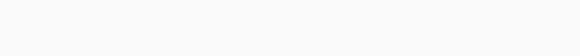# Solved – Clarifications regarding reading a nomogram

Following is a nomogram created from mtcars dataset with rms package for the formula:

``mpg ~ wt + am + qsec ``The model itself seems good with R2 of 0.85 and P<0.00001

``> mod  Linear Regression Model  ols(formula = mpg ~ wt + am + qsec, data = mtcars)                  Model Likelihood     Discrimination                        Ratio Test           Indexes         Obs       32    LR chi2     60.64    R2       0.850     sigma 2.4588    d.f.            3    R2 adj   0.834     d.f.      28    Pr(> chi2) 0.0000    g        6.456      Residuals      Min      1Q  Median      3Q     Max  -3.4811 -1.5555 -0.7257  1.4110  4.6610             Coef    S.E.   t     Pr(>|t|) Intercept  9.6178 6.9596  1.38 0.1779   wt        -3.9165 0.7112 -5.51 <0.0001  am         2.9358 1.4109  2.08 0.0467   qsec       1.2259 0.2887  4.25 0.0002   ``

I am not clear what are these 'Points', 'Total Points' and 'Linear Predictor'. Which one of these represent mpg, the outcome variable? I will appreciate any explanation.

Edit: considering excellent suggestions by @Glen_b for easy reading of points etc, following could be an alternative nomogram:Since outcome or response variable is available, it can be used instead of the term 'Linear Predictor'. It also become self-explanatory as to how the nomogram needs to be read.

Contents

Well, since your model is linear, with the expected mpg equal to the linear predictor, you can read mpg straight off the linear predictor scale.

For each variable, you find its value on the relevant scale. For example, imagine we wanted to find a predicted mpg for a car with `wt=4, am=1, qsec=18`:which gives a predicted mpg of about 18.94. Substituting into the equation gives 18.95, so that's pretty close. (In practice you would probably only work to the nearest whole point — and so get about 2 figure accuracy – "19 mpg" – out, rather than 3-4 figures as here.)

One of the chief benefits of such a diagram to my mind is that you instantly see the relative effect of changes in the different predictor variables (IV) on the response (DV). Even when you don't need the diagram for any calculations, it can have great value in terms of simply displaying the relative effects of the variables.

Does it work the same way for non-linear or polynomial regressions?

For cases where \$E(Y)\$ is nonlinear in some predictors, some minor – and perhaps obvious – modifications are needed. Imagine that we have \$hat{y} = b_0+b x_1+f(x_2)\$

where either:

(a) \$f\$ is monotonic; or

(b) \$f\$ is not monotonic

In either case, the scale for \$x_1\$ would work exactly as above, but in case:

(a) the scale for \$x_2\$ won't be linear; e.g. if \$f\$ is monotonic decreasing but (roughly) quadratic, you might have something like this:(b) the non-monotonic scale for \$x_2\$ will "break" at a turning point and flip over. e.g.— here the function \$f(x)\$ has a minimum somewhere around \$x=2.23\$

It's possible for such functions to have several turning points, where scales would break and flip over multiple times – but the axis line only has two sides.

With points-type nomograms this presents no difficulty, since one may move additional scale-sections up or down (or more generally, orthogonally to the direction of the axis) a little until no overlap occurs.

(More than one turning point can be a problem for alignment-type nomograms; one solution shown in Harrell's book is to offset all the scales slightly from a reference line, on which the value's position is actually taken.)

In the case of GLMs with nonlinear link function, the scales work as above, but the scale of the linear predictor will be marked with a nonlinear scale for \$Y\$, something like (a) above.

Examples of all of these situations can be found in Harrell's Regression Modeling Strategies.

Just a couple of side notes

1. I'd much prefer to see two points scales, at the top and bottom of the relevant section; otherwise it's hard to "line up" accurately because you have to guess what 'vertical' is. Something like this:However, as I note in comments, for the last section of the diagram (total points and linear predictor) perhaps a better alternative to a second points scale would be to simply have a pair of back-to-back scales (total points on one side, linear predictor on the other), like this:whereupon we avoid the need to know what 'vertical' is.

2. With only two continuous predictors and a single binary factor, we can quite readily construct a more traditional alignment nomogram:In this case you simply find the `wt` and `qsec` values on their scales and join them with a line; where they cross the `mpg` axis, we read off the value (while the `am` variable determines which side of the `mpg` axis you read). In a simple case like this, these kind of nomograms are faster and simpler to use, but can be less easy to generalize to many predictors, where they can become unwieldy. The points-style nomogram in your question (as implemented in Regression Modeling Strategies and in the `rms` package in R) can add more variables seamlessly. This can be quite an advantage when dealing with interactions.

Rate this post